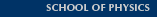# Heisenberg's uncertainty principle and the musician's uncertainty principle

Heisenberg's Uncertainty Principle follows from a classical result, which is at least as old as Fourier. We prefer to introduce it as the Musician's Uncertainty Principle. This page explains the classical result and uses sound files to demonstrate it. It then shows how the Heisenberg's Uncertainty Principle follows directly from the classical observation, plus the observation of quantisation.

When musicians tune up, we listen to the note for a long time so that we can adjust the frequency precisely. We tune by removing beats, which are regular pulsations of loudness produced by notes that are nearly in tune. (See What are interference beats?) If the frequency difference is one hertz (one vibration per second), then you hear an interference beat every second (demonstrated below). If the frequency difference is two hertz, then you hear two interference beats per second: the difference between the frequencies of the interfering sounds equals the number of beats per second.

How long do you need to determine whether two notes are in tune? Let's find out experimentally. Download these sound files and listen, starting with the shortest file and continuing until you can hear beats.

 400 & 403 Hz 0.08 s0.17 s0.33 s0.67 s1.00 s2.00 s5.00 s400 & 401 Hz      Sound files courtesy John Tann 0.08 s0.17 s0.33 s0.67 s1.00 s2.00 s5.00 sYour results might depend upon your hearing, how much background noise there is, and how hard you concentrate. But you probably found that, when the frequencies differed by 3 Hz, you needed (very roughly) about a third of a second. When they differed by 1 Hz, you needed more time. So, roughly speaking, if the frequencies differ by Δf, then you need a time of 1/Δf to notice. In other words:

Δf.Δt > ~ 1     or, in non-mathematical language:
(time taken to measure f) times (error in f) is on the order of one, or larger. This result was known to Joseph Fourier In this context, I call it:

The musician's uncertainty principle. Because musicians know this, qualitatively at least. If the chord is short, or if you are playing a percussive instrument, the tuning is less critical. In a long sustained chord, you have to get the tuning accurate. And of course oboists in orchestras play notes for tens of seconds while the other instruments tune carefully before a concert.

So far this calculation is just an order-of magnitude. One can imagine doing a litte better than  Δf.Δt > 1  by careful measurement. (Have a look at the diagrams on What are interference beats?). The uncertainty principle is usually written with an extra factor of 2π: it takes about one radian rather than a whole cycle. So:

(time taken to measure f) times (error in f) is greater than about 1/2π.

### Heisenberg's uncertainty principle

Now in quantum mechanics and atomic physics, the energy of a photon is hf, where h is Planck's constant. So a measurement of the energy corresponds to a measurement of the frequency, and that, as we have seen, takes time. Mutliplying our previous inequality by h on both sides gives us Heisenberg's uncertainty principle for energy
(uncertainty in energy) times (uncertainty in time) is greater than about h/2π, or

ΔE.Δt > ~ h/2π.

Heisenberg's uncertainty principle for momentum is analogous. Let's consider the spatial frequency F (which is defined as the number of cycles per unit distance) rather than temporal frequency (number of cycles per unit time). F is just the reciprocal of the wavelength, λ. The same argument about beats in this case gives (for motion in the x direction)

ΔF.Δx = Δ(1/λ).Δx > ~ 1
The momentum of a photon (or anything else) is p = h/λ so, multiplying the above equation by h gives
Δp.Δx > ~ 1
which is usually written as
Δpx.Δx > ~ h/2π.

(uncertainty in momentum) times (uncertainty in position) is greater than h/2π.

Werner Heisenberg won the Nobel prize in 1932.

Practical consequences of the uncertainty principle. h is very small (6.63 10-34 Js), so the consequences of the uncertainty principle are usually only important for photons, fundamental particles and phonons. (See, for example, this example using a cricket ball.) There are, however, many physical processes whose evolution with time depends sensitively on the initial conditions. (Sensitivity to intial conditions is fashionably called chaos.) The uncertainty principle prohibits exact knowledge of initial conditions, and therefore repeated performances of such processes will diverge. Physicists will also tell you that one cannot have exact knowledge anyway, for a variety of practical reasons, including the fact that you don't have enough memory to record the infinite number of significant figures required to record an exact measurement.

There is also a discussion of how chemistry depends upon the uncertainty principle at Why there would be no chemistry without relativity, which is part of our site on relativity.

Philosophical consequences of the uncertainty principle. Some philosophers regard the consequences of the uncertainty principle as having a more fundamental importance. Their argument goes like this: if one could know exactly the position, velocity and other details, one could, in principle, compute the complete future of the universe. Since one cannot know the position and momentum of even one particle with complete precision, this calculation is impossible, even in principle. Some attempt to draw profound conclusions from this simple observation. Most scientists find this a trivial argument. A memory capable of storing all this information would be as complex as the universe, and then the contents of that memory would have to be included in the calculation, and that would make the amount of information greater, and that information would have to be stored..... We rather point out that all of that information is actually contained in the universe which, as an analogue computer, is computing its own future already.[Basics | Research | Publications | Flutes | Clarinet | Saxophone | Brass | Didjeridu | Guitar | Violin | Voice | Cochlear ]
[ People | Contact Us | Home ]

© Joe Wolfe / J.Wolfe@unsw.edu.au
phone 61-2-9385 4954 (UT + 10, +11 Oct-Mar)
Joe's music site